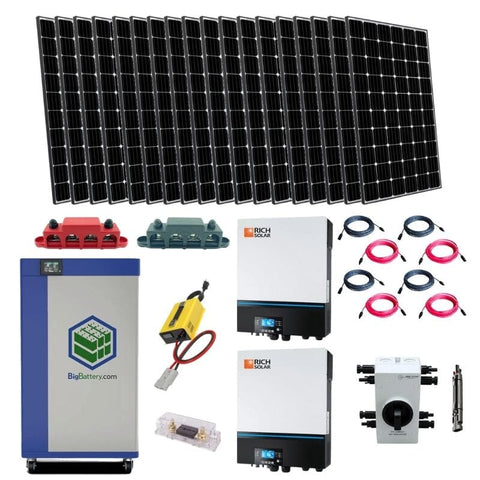Convert MWh to KWh - A Breakdown of the Basics - ShopSolar.com

# Convert MWh to kWh

Units like megawatt-hours and kilowatt-hours can seem almost too big to comprehend when you’re first setting food in the world of electrical equations. However, once you take an entire household’s electrical usage into account, you quickly run into this territory, and being able to convert MWh to kWh becomes essential.

If we only have access to one of the variables written in a particular way, we need to know how to convert it quickly so that we can figure out what we’re dealing with.

For example, most household electricity bills are written in kWh. So if we only have MWh to work with, we need to be able to work it out to make sure everything lines up.

Let’s look at how to convert MWh to kWh and discover their significance in the world of solar.

## What Are MWh?

When we look at something like a milliamps-to-amps calculator, it’s taking the same unit of power, but increasing the scale. Today’s conversion is a similar process.Before we get down to the differences between the variables, we need to introduce the topic of power.

Power is the rate at which energy is transferred and it’s measured in watts.

1,000 watts (W) is equivalent to 1 kilowatt (kW) and 1 megawatt (MW) is equivalent to 1,000 kilowatts. In other words, these are massive amounts of power that we’re dealing with.

Let’s look at a more practical example to get an understanding of how this works.

### Example

We’ve used a farm or house amp calculator to see which appliances are going to be the most power-hungry when hooked up to our solar system.

From there, we’ve set up an array of portable, flexible solar panels connected to some deep-cycle solar batteries that generate 10 MW.The maximum output for this solar system when only looking at the solar panels is 10 MW. So what are megawatt hours?

These are the units used to measure energy over time. In other words, 1 megawatt-hour (MWh) means that a single megawatt is sustained for 1 hour. This counts for both energy generation and consumption.

This is also the value you should look at when deciding on the size of your solar battery, since you want to build up energy to use for when the sun isn’t shining and your solar array isn’t producing at its maximum capacity.

Megawatt hours are a large amount of energy so it might be a bit overkill for those who have their requirements after using an RV amp calculator.

RVs typically require energy on a kilowatt or watt scale. Megawatt-sized arrays cater more towards commercial or industrial use.

## What Are kWh?

Kilowatt-hours are going to look familiar if you’ve seen your monthly power bill. Before we dive into that, we should look at kilowatts on their own.

The kilowatts consumption of an appliance can normally be seen on a sticker, telling you how much energy per second the appliance uses.Kilowatt-hours is the amount of power that’s demanded from these appliances over an hour. When we multiply power by time, we get energy.

There are instances where you won’t have this value and it needs to be calculated.

It might not follow the same units of power when you convert volts to amps, but the simplicity of the conversion remains.

### Example

Let’s say we have an air conditioning unit connected to our solar system. It’s rated at 4 kW and we want to run it for 4 hours.

Calculating the kilowatt-hours is simply a matter of multiplying the kW rating by the time. This means the energy consumption of the AC unit will be 16 kWh.

So now that we understand the variables, let’s see how we would convert them.

## Converting MWh to kWh

The conversion process is like using a mAh-to-amps calculator.

1 MWh is equivalent to 1,000 W. So if you’re wanting to know how to convert 3.25 MWh to kWh, simply multiply the megawatts by 1,000 to end up with 3,250 kWh.## Conclusion

Converting MWh to kWh isn’t the problem as it's mostly dividing or multiplying by 1,000.

When we break down the variables as individuals, we can understand them. This makes converting them second nature.

Did You Find Our Blog Helpful? Then Consider Checking: